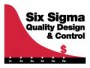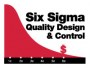# Six Sigma Metrics

In 7 lessons, you will learn the theory, tools and applications of analytical Sigma Metrics.
Base price with tax
Salesprice with discount
\$195.00
Discount
Tax amount
Price / kg:Description

In 7 lessons, you will learn the theory, tools and applications of analytical Sigma Metrics.

 Course Lesson 1. Introduction to Six Sigma In this lesson, we introduce the concepts behind Six Sigma, the history of the development of this quality management technique, and highlight applications of Six Sigma in the healthcare field. We will review the idea of Six Sigma quality management; Define the "Six" in Six Sigma; Understand the meaning of "Defects per Million Opportunities (DPMO)“; and Evaluate and compare different processes in and out of healthcare using Defects Per Million counting. 2. Two Approaches to Six Sigma In this lesson, you will learn there are actually two ways to determine a Sigma-metric. The most common approach is to count defects, determine the defect%, converted to a defects-per-million rate, and then use a table to look up the appropriate Sigma-metric. The second approach is to use the measurement of process variation to estimate the Sigma-metric. By the end of this lesson, you will be able to Identify the two approaches to determining Sigma-metrics; Learn when the counting approach is more appropriate; Learn when the calculation approach is more appropriate; Compare some common, known Sigma metrics to less well-known metrics of the laboratory 3. Analytical Performance Capability In this lesson, we encounter the equations that determine the analytical Sigma-metric, and how this metric is related to short-term Sigma metric. We introduce the concepts behind Six Sigma, the history of the development of this quality management technique, and highlight applications of Six Sigma in the healthcare field. By the end of this lesson, users will discern the difference between the Six Sigma assessment of discrete vs continuous variables; Define the Sigma-metric equation and all the variables within it; Derive the precision requirements necessary to achieve CLIA goals with Six Sigma reliability; Compare the Cpk metrics with the Six Sigma metrics 4. QC Design and Rule Selection In this lesson, we move beyond just the calculation of the Sigma-metric. We explore its implications - what does it mean to have a Six Sigma method? What does it mean to have a Three Sigma method? What are the consequences to laboratory operation? How many controls must be run? How many rules must be implemented and interpreted? How often must the lab run QC for any particular method? By the end of this lesson, participants will understand the derivation of the Sigma-metric from the Critical Systematic Error; Construct, use and interpret results on a Method Decision Chart; Construct, use and interpret results on an OPSPecs chart; Differentiate between error detection and false rejection in QC procedures 5. Quality Design and Control Selection In this lesson, we concentrate on the implementation of the Six Sigma metric implications. There are multiple variables that are used to determine the Sigma-metric. There are also multiple factors that determine the performance of a QC procedure. All of those variables and factors combine on an OPSpecs chart to determine what QC procedure is most appropriate for a particular laboratory method. There are multiple applications for the OPSpecs chart, from setting goals for a method purchase, to optimizing QC for the method that you have purchased. By the end of this lesson, participants will determine analytical and/or clinical quality performance specifications for a test; outline the steps for setting purchase performance specifications; outline the steps for choosing the most appropriate QC procedure 6. Using Method Decision Charts and OPSpecs Charts In this lesson, we drill down to great detail of the construction and use of the Method Decision Chart and the OPSpecs chart. These graphs encapsulate all of the complexity of QC procedure capability and Six Sigma performance. Rather than calculate equations, the user can simply make visual judgments. These tools are used to speed the interpretation of method acceptability and QC optimization. By the end of the lesson, participants will understand how to build a Method Decision Chart; understand how an OPSpecs chart is constructed; plot the operating point of a test method on both a Method Decision Chart and an OPSpecs chart; understand how to select the best QC procedure for a method on an OPSpecs chart 7. Advanced tools for Six Sigma Application In this final lesson, we describe the advanced tools for Six Sigma metrics. Normalized Method Decision Charts and Normalized OPSpecs charts allow a laboratory to display the performance of multiple methods on a single convenient chart. "Westgard Sigma Rules" is a very simplified assessment and design tool - allowing a single simple tool to assess and redesign QC. "Westgard Sigma Rules" with QC Frequency Recommendations harnesses the additional power of the MaxE(nuf) model to determine the best QC frequency (run size) depending on the Sigma-metric of the method. Objectives: By the end of this lesson, participants will understand how to build a Method Decision Chart; understand how an OPSpecs chart is constructed; plot the operating point of a test method on both a Method Decision Chart and an OPSpecs chart; understand how to select the best QC procedure for a method on an OPSpecs chart; understand how to modify QC frequency based on Sigma-metrics.
Joomla SEF URLs by Artio